# Python Variable Scope

• Python local variables with an example
• Python nonlocal keyword with an example
• Python global variables with an example

## Python Local Variables

All variables that are declared inside a functionclass are local variables. Or you can say that a variable inside a function or class is considered a local variable only if it appears on the left side of an assignment statement (=, +=, -=, etc.). For example, if you found any variable in a way like a = 5 or for a in range (5) or some other binding, then the variable a is said to be a local variable.

Local variables belong to the local scope. That is, in the program given below:

```def codescracker():
num = 2
print(num)

codescracker()```

The scope of the "num" variable is only for the function where it is defined. That is, outside of the function (codescracker), the variable num is unknown or undefined. The above program produces 2 as an output.

Now a question may arise in your mind: What if we use a local variable outside the function? Therefore, I've created a program, as given below, that uses a local variable outside the function:

```def codescracker():
num = 2

print(num)```

The above program produces an error (NameError) like shown in the snapshot given below: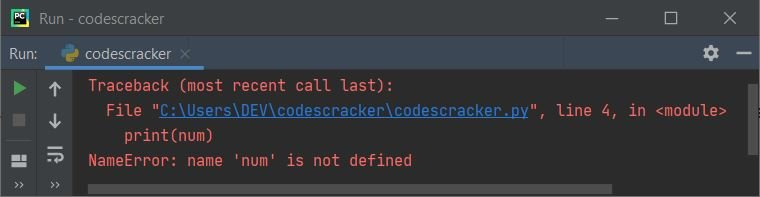Now we may have another question in our minds: what if we want to access a variable created locally all over the program? The answer to this question is global keyword. You'll learn about global variables later on in this tutorial. Now let's take a look at the example given below of a local variable in Python:

```def codescracker():
val = 10
print("Value of variable \"val\" is", val)

val = 5
print("Value of variable \"val\" is", val)
codescracker()
print("Value of variable \"val\" is still", val)```

The snapshot given below shows the sample output produced by the above program:As you can see from the above example, the variable val with a value of 5 is a global variable, and the same variable declared inside the function codescracker() is considered a local variable. Therefore the value 10 is initialized to it, is local and holds only until the execution of that function.That is, when you exit from that function, the value 10 gets lost, and 5 will be the original value of variable val.

## Python nonlocal keyword

Since Python does not allow us to reassign a new value to a variable from the outer scope to the inner scope, Therefore, to overcome this problem, Python provides a keyword named nonlocal. This keyword is used when we need to perform any assignment operation on a variable that is already declared inside the outer function. For example, consider the following Python program:

```def outer_fun():
num = 10
def inner_fun():
num = num + 2
print(num)
inner_fun()

outer_fun()```

produces an UnboundLocalError like shown in the snapshot given below: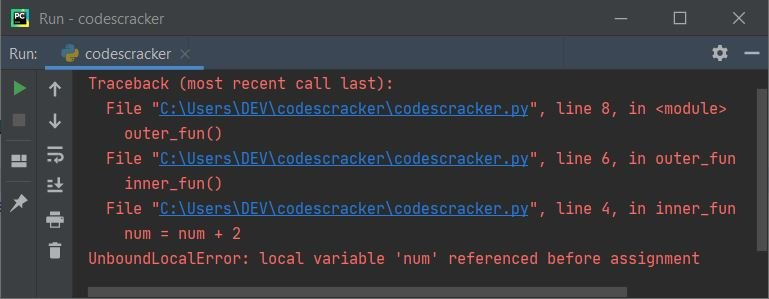This is because if there is an assignment to a variable inside a function, then that variable is considered a local variable. Therefore, we must have that variable created using the nonlocal keyword as shown in the program given below:

```def outer_fun():
num = 10
def inner_fun():
nonlocal num
num = num + 2
print(num)
inner_fun()

outer_fun()```

This time, the output produced looks like this: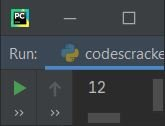That is, 12 is the output produced by the above program without producing any errors. However, if you do not need to assign anything to the variable num (a previously created variable), you can continue without further declaring the variable, as shown in the program below:

```def outer_fun():
num = 10
def inner_fun():
print(num)
inner_fun()

outer_fun()```

The output produced by this program is 10, not 12, because I haven't increased its value by 2 as I did in the previous program.

Note: If you found any nonlocal variables, then these variables belong to an enclosing function. That is neither said to be local nor global.

## Python global variables

A global variable can be created in Python in two ways. The first method is to define variables outside of all functions and classes, i.e. outside of the main program. And the second way is to define a variable anywhere in the program using the global keyword. Here is an example of a Python global variable:

```def codescracker():
global val
val = 10
print("Value of variable \"val\" is", val)

val = 5
print("Value of variable \"val\" is", val)
codescracker()
print("Now the Value of variable \"val\" is", val)```

The snapshot given below was taken from the output produced by the above Python program: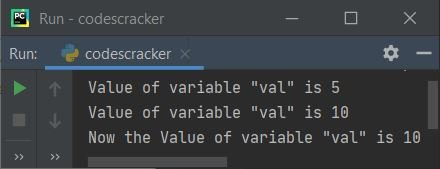Here is another example of creating two global variables, one using the normal way (inside the main program) and the second using the global keyword as told above:

```num = 10

def codes():
print(num)

def cracker():
global val
val = 12
print(val)

def codescracker():
print(val)

print(num)
codes()
cracker()
print(val)
codescracker()```

The output produced by the above program is: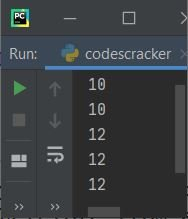The above program works in such a way that:

• First, a variable "num" is created and initialized with 10.
• Since this variable is created outside of any function or class, its scope is global.
• Because the variable has a global scope, we can access it from any function or class.
• I've defined two methods named "codes()" and "cracker()". But the program flow goes to the "print(num)" statement (that is outside both functions), after executing the first statement, which was "num = 10."
• The value of "num" equal to 10 is printed on output using the "print(num)" statement.
• Now the statement "codes()" gets executed.
• That is, the method "codes()" gets called, and inside this method, a statement, "print(num)," gets executed.
• Since "num" is globally defined, the value of "num" gets printed on the output from inside the method named "codes."
• Now the method "cracker()" gets called. Inside this method, a variable named "val" gets defined using the "global" keyword.
• Since the variable is defined using the "global" keyword, it can be accessed from anywhere in the whole program.
• Now 12 gets assigned and printed using the "print(val)" statement.
• And at second last, the "print(val)" statement gets executed, which prints 12 on the output.
• It should be noted that the second last statement accessed a variable "val" defined within the "cracker()" method.This is happening because I've used the "global" keyword to define the variable.
• And since the variable "val" is defined with the "global" keyword, it can also be accessed from within another function, as shown in the above program. That is, "codescracker()" also gets access to the variable "val."

### Python Local vs. Global Variable Example Program

This is the example that you need to understand. After understanding this program, I'm sure you'll get a complete understanding of local and global variables in Python.

```val = 50

print("\nI am inside the local scope of read_from_global().")
print("Value of the variable \"val\" is",val)

def local():
val = 100
print("\nI am inside the local scope of local().")
print("Value of the variable \"val\" is",val)

def change_global_variable():
global val
val = 100
print("\nI am inside the local scope of change_global_variable().")
print("Value of the variable \"val\" is",val)

print("\nI am in the global scope.")
print("Value of the variable \"val\" is",val)

print("\nI'm back in the global scope.")
print("Value of the variable \"val\" is still",val)

local()
print("\nI'm again back in the global scope.")
print("Value of the variable \"val\" is still",val)

change_global_variable()
print("\nI'm again back in the global scope.")
print("Now this time, the value of the variable val becomes",val)```

Here is its sample output: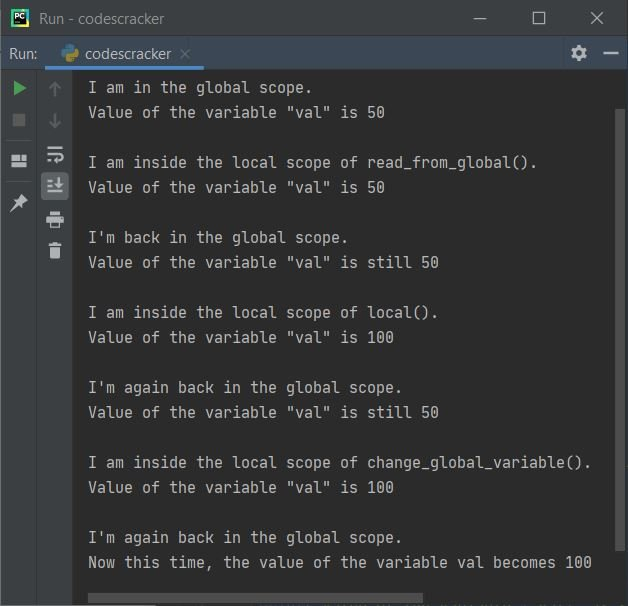## Python Variable Scope Example

This example program may clear all your remaining doubts (if any) about local and global variables along with the nonlocal keyword:

```num = 10

def codes():
num = 200
print("2. ",num)
def inner():
nonlocal num
num = num + 50
print("3. ",num)
inner()
print("4. ",num)

def cracker():
global val
val = 20
print("5. ",val)
print("6. ",num)

def codescracker():
print("7. ",val)

print("1. ",num)
codes()
cracker()
codescracker()
print("8. ",val)```

The number is provided for each print statement; that shows the serial number that the print statement gets executed. The snapshot given below, taken from the output produced by the above program, reads as follows: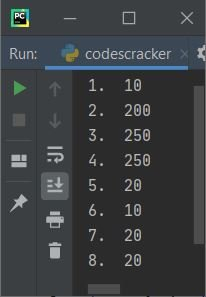Take a deep look at the above program. It could take a few minutes. But I'm sure that it will provide you everything about variable scope in Python.

Python Online Test

« Previous Tutorial Next Tutorial »